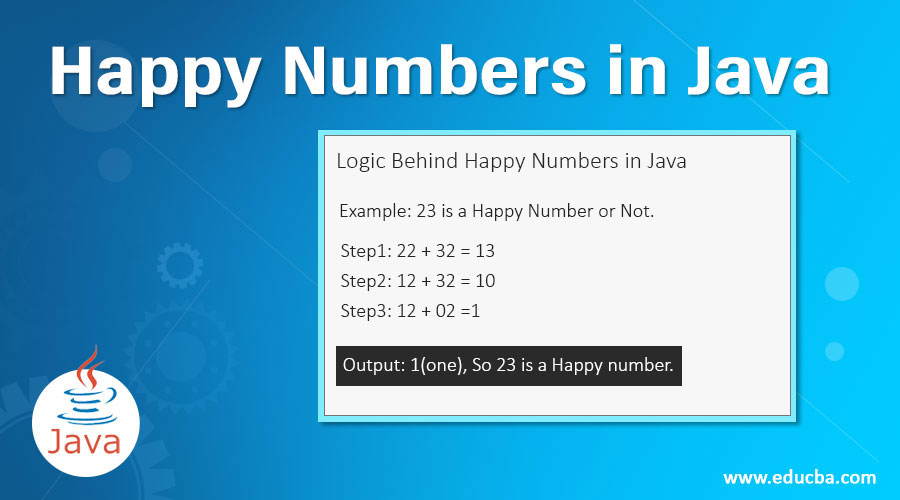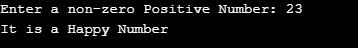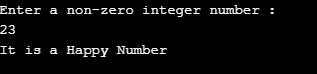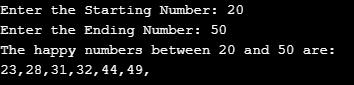# Happy Numbers in Java

Tags: number## Introduction to Happy Numbers in Java

Happy Number are positive non-zero integer numbers. If we find the sum of the squares of its every digit and repeat that process until the number will be equals to 1(one). Otherwise, it is called Unhappy Number or Sad Number. So, in this article, we will discuss happy numbers in java in detail.

The examples of Happy Numbers are: 1, 7, 10, 13, 19, 23, 28, 31, 32, etc.

Logic Behind Happy Numbers in Java:

 Example: 23 is a Happy Number or Not. Example: 11 is a Happy Number or Not. Step1: 22 + 32 = 13 Step1: 12 + 12 = 2 Step2: 12 + 32 = 10 Step2: 22 = 4 Step3: 12 + 02 =1 Output: 1(one), So 23 is a Happy number. Output: 4(four), So 11 is an Unhappy number.

### Algorithm to Find Happy Numbers in Java

Following are the different steps to find happy numbers in java.

Step 1: To Enter a non-zero, positive number from keyboard and assign to the variable is called number.

Step 2: To calculate the remainder by dividing (%) the given number with 10.

Step 3: Calculate the square of the remainder value and add it to a variable sum.

Step 4: To divide (/) the number by 10.

Step 5: Repeat step: 2 to step: 4, until we get the sum of the square of all digits of the given number.

Step 6: Then the final addition value stored in the variable sum.

Step 7: To define a variable called result and initialize it with the value of a number.

Step 8: If the result value is not equal to 1 or 4 then, we will simply call the created method to repeat the process.

Step 9: If the result value set to 1 then, it prints” it is a Happy Number “else it prints “It is not a Happy Number”.

Note:

• In Happy Number, the number is not affected by inserting/deletion Zeros on any side.
• If one number will be Happy Number i.e. a sequence of a number is happy. For Example, 23 is a Happy Number; it indicates the sequence of numbers like 13,10,1 are should be Happy Number.
• If finally, the sum of the squares of its digit equals to 4(four) i.e. it is Unhappy.

### Examples to Check Happy Numbers in Java

Following are the different examples to check happy numbers in Java.

#### Example #1

We create a checkHappyNumber ( ) method to verify the given number will be a Happy Number or Not.

Code:

```import java.util.*; public class HappyNumber { public static int checkHappyNumber (int number) { int rem = 0, sum = 0; // calculate the sum of squares of each digits while(number > 0) { rem = number %10; sum = sum+(rem*rem); number = number/10; } return sum; } public static void main(String[] args) { // Take number from KeyBoard Scanner sc = new Scanner (System.in); System.out.print("Enter a non-zero Positive Number:"); int number = sc.nextInt( ); int result = number; while (result != 1 && result != 4) { result = checkHappyNumber(result); } if (result ==1) { System.out.println ("It is a Happy Number"); } else { System.out.println (" It is not a Happy Number"); } } }```

Output:#### Example #2

We create a checkHappyNumber ( ) method to verify the given number will be a Happy Number or Not.

Code:

```import java.util. *; public class Main { public static Boolean checkHappyNumber(int number) { Set digits=new HashSet(); while(digits.add(number)) { int result = 0; while(number > 0) { result += Math.pow(number % 10, 2); number = number/10; } number = result; } return number == 1; } public static void main(String[] args) { Scanner sc = new Scanner(System.in); System.out.println ("Enter a non-zero integer number :"); int number = sc.nextInt(); System.out.println(checkHappyNumber(number)?"It is a Happy Number":"It is an Unhappy Number"); } }```

Output:#### Example #3

We create a checkHappyNumber ( ) method to verify all the numbers between a range of numbers are Happy Number or Not and also print the list of Happy Numbers.

Code:

```import java.util.*; public class HappyNumber { public static int checkHappyNumber(int number) { int rem = 0,sum = 0; // calculate the sum of squares of digits while(number >0) { rem = number%10; sum = sum + (rem*rem); number = number/10; } return sum; } public static void main(String[] args) { // Take starting and ending number from keyboard Scanner sc = new Scanner(System.in); System.out.print("Enter the Starting Number:"); int i=sc.nextInt(); System.out.print("Enter the Ending Number:"); int j=sc.nextInt(); System.out.println("The happy numbers between "+i+" and "+j+" are: "); for (int x=i ; x { int result = x; //Happy number always ends with 1 and unhappy number ends with 4 while(result != 1 && result != 4) { result = checkHappyNumber(result); } if(result == 1) System.out.print(x + ","); } } }```

Output:### Conclusion

In this article, we will specially discuss with Happy Numbers in Java. It is a fascinating number. Where the sum of squares of each digit of the number and repeat the same process to get finally 1. After compilation of all process if the result is not equal to 1 or equal to 4, is called Unhappy Number or Sad Number. Like happy numbers, if a number is unhappy, then all of its sequence members are also unhappy numbers. We provide different java programs related to checking Happy Numbers by using different methods in java.

### Recommended Articles

This is a guide to Happy Numbers in Java. Here we discuss the Introduction and algorithm to find Happy Numbers in Java along with examples and code implementation. You may also look at the following articles to learn more –

1. Examples of Prime Numbers in Java
2. Various Methods of Java LocalDateTime
3. How Single Inheritance Works in Java?
4. Implementation of Native Methods in Java

The post Happy Numbers in Java appeared first on EDUCBA.

This post first appeared on Free Online CFA Calculator Training Course | EduCB, please read the originial post: here